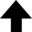Logic is the cement of our civilization with which we ascend from chaos using reason as our guide.T'Plana-Hath, Matron of Vulcan philosophy

What is the density effect correction to the Bethe-Bloch formula? — The density effect can be qualitatively derived in classical electrodynamics [J.D. Jackson, Classical Electrodynamics, 2nd ed., John Wiley, New York, 1975]. In dense media, the field which perturbs electrons far from the projectile track is modified by the dielectric polarization of the atoms between the distant electron and the projectile. The magnitude of the density effect was originally calculated by Fermi [E. Fermi, "The Ionization Loss of Energy in Gases and in Condensed Materials," Phys. Rev. 57, 485 (1940)] and extended by Sternheimer and Peierls [R.M. Sternheimer and R.F. Peierls, "General Expression for the Density Effect for the Ionization Loss of Charged Particles," Phys. Rev. B 3, 3681 (1971)].

If you give this program a chemical (empirical) formula and its corresponding mass density $\rho$ (in g/cm3), it will return the Sternheimer density effect parameters of total atomic charge $Z$, total atomic mass $A$, mean ionization potential $I$ (in eV), plasma frequency $\omega_\text{p}$ (in eV), $X_0$, $X_1$, $a$, $m$, $\delta_0$, gas density ratio $\eta$, and the total electronic binding energy $E$ (in keV). It uses the prescription given in "Evaluation of the collision stopping power of elements and compounds for electrons and positrons," Int. J. Appl. Radiat. Isot. 33(11), 1189 (1982) by Stephen M. Seltzer and Martin J. Berger.

## Density Effect Correction to the Bethe-Bloch Formula

This program calculates the Sternheimer density effect parameters using the prescription given in The International Journal of Applied Radiation and Isotopes 33(11), 1189 (1982). This utility is a companion to the Range-Energy Calculator.
 1-H 2-He 3-Li 4-Be 5-B 6-C 7-N 8-O 9-F 10-Ne 11-Na 12-Mg 13-Al 14-Si 15-P 16-S 17-Cl 18-Ar 19-K 20-Ca 21-Sc 22-Ti 23-V 24-Cr 25-Mn 26-Fe 27-Co 28-Ni 29-Cu 30-Zn 31-Ga 32-Ge 33-As 34-Se 35-Br 36-Kr 37-Rb 38-Sr 39-Y 40-Zr 41-Nb 42-Mo 43-Tc 44-Ru 45-Rh 46-Pd 47-Ag 48-Cd 49-In 50-Sn 51-Sb 52-Te 53-I 54-Xe 55-Cs 56-Ba 57-La 58-Ce 59-Pr 60-Nd 61-Pm 62-Sm 63-Eu 64-Gd 65-Tb 66-Dy 67-Ho 68-Er 69-Tm 70-Yb 71-Lu 72-Hf 73-Ta 74-W 75-Re 76-Os 77-Ir 78-Pt 79-Au 80-Hg 81-Tl 82-Pb 83-Bi 84-Po 85-At 86-Rn 87-Fr 88-Ra 89-Ac 90-Th 91-Pa 92-U 93-Np 94-Pu 95-Am 96-Cm 97-Bk 98-Cf 99-Es 100-Fm
 Mass density $\rho$: g/cm3 The material is a gas
Chemical formula:
Total atomic charge $Z$:
Total atomic mass $A$:
Mean ionization potential $I$: eV
Mass density $\rho$: g/cm3
Plasma frequency $\omega_\text{p}$: eV
$X_0 =$
$X_1 =$
$a =$
$m =$
$\delta_0 =$
Gas density ratio $\eta$:
Total electronic binding energy $E$: keV
Reset calculator